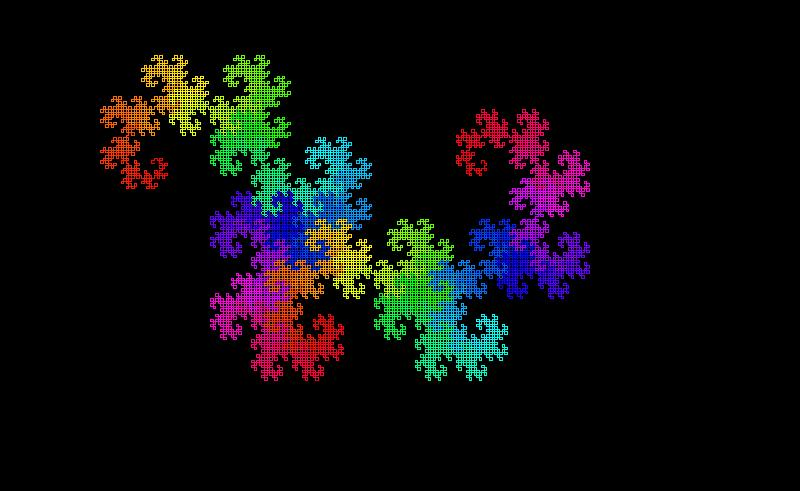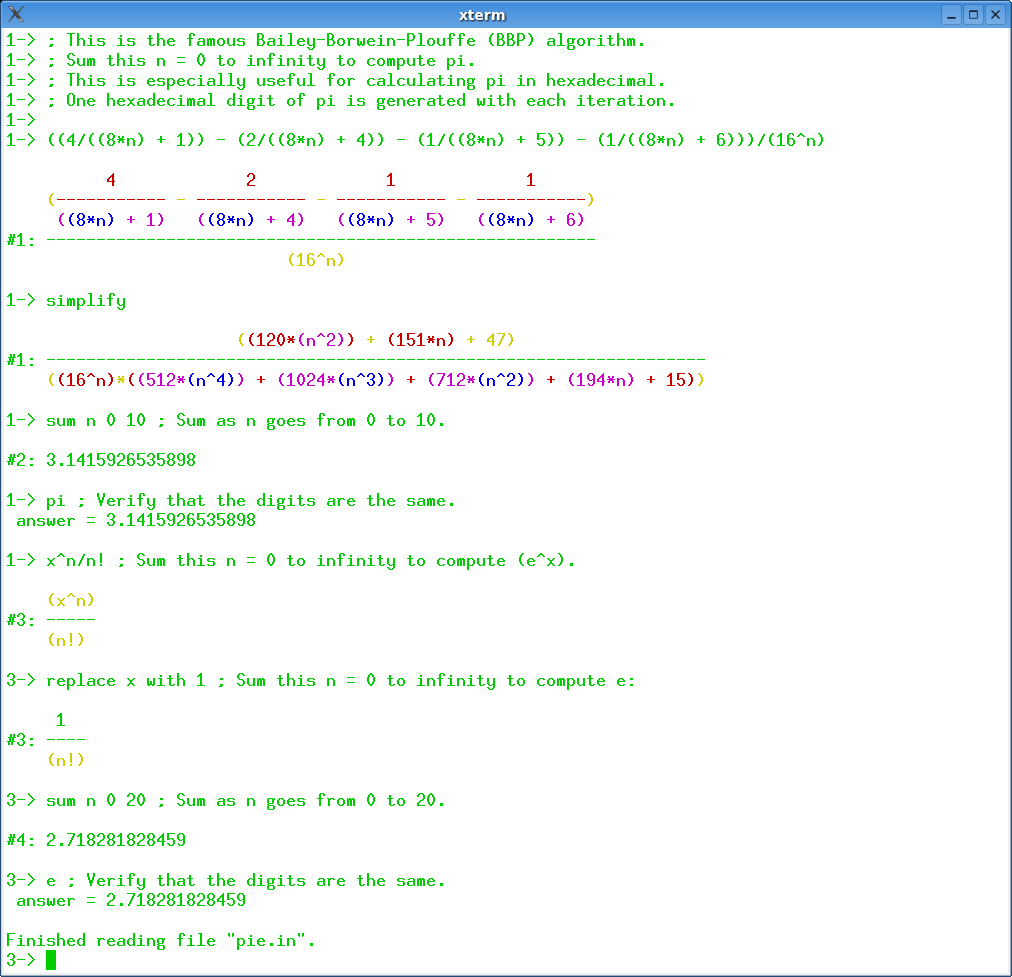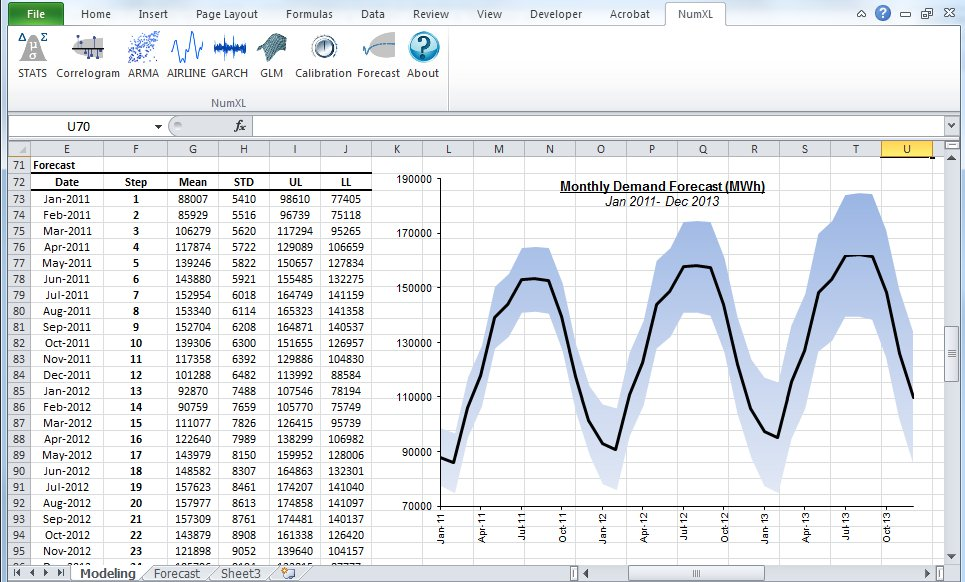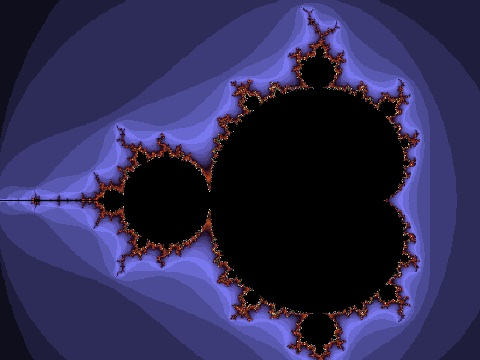## 34 Search Results

### 3D-XplorMath

The primary goal of 3D-XplorMath is to allow users with little or no programming experience to see, with minimal effort, concrete visual representations of many different categories of mathematical objects and processes. To accomplish this, objects from each category are described internally by well-designed, parameterized data structures, and for each category a variety of rendering methods is provided to permit visualization of objects of the category in ways that are appropriate for various purposes. Each of the hundreds of built-in objects known to the program is assigned carefully chosen defaults so that, when the object is selected from a menu, the program can immediately construct a standard example of the object and render it in an optimized view. The user may then use various menus and dialogs to alter the parameters describing the shape and coloration of the object, change the viewpoint from which it is seen, select different rendering methods, etc. Moreover, as its name suggests, the program can display objects such as surfaces, space curves and polyhedra using various stereo techniques. In addition to the many built-in objects known to the program, a user can create "user-defined" objects by entering formulas using standard mathematical notation. Visualizations created by the program can be saved in jpeg and other graphic formats and the data defining 3D objects can be exported to other 3D programs (e.g., Bryce or POV-Ray) in formats such as .obj and .inc. Both built-in and user-defined objects can depend on parameters, and the program can create morphing animations by moving along a path in the parameter space, and these animations can then be saved as QuickTime movies. Each of the built-in objects has associated to it a so-called ATO (About This Object) file that provides documentation for the object. An early and more developed version of the program, written in Object Pascal, runs under the Macintosh Operating System and a Java-based cross-platform version is now also available.

More information### CASA

CASA is a special-purpose system for computational algebra and constructive algebraic geometry. The system has been developed since 1990. CASA is the ongoing product of the Computer Algebra Group at the Research Institute for Symbolic Computation (RISC-Linz), the University of Linz, Austria, under the direction of Prof. Winkler. The system is built on the kernel of the widely used computer algebra system Maple.

### CGAL

CGAL is a collaborative effort of several sites in Europe and Israel. The goal is to make the most important of the solutions and methods developed in computational geometry available to users in industry and academia in a C++ library. The goal is to provide easy access to useful, reliable geometric algorithms The CGAL library contains: the Kernel with geometric primitives such as points, vectors, lines, predicates for testing things such as relative positions of points, and operations such as intersections and distance calculation, the Basic Library which is a collection of standard data structures and geometric algorithms, such as convex hull in 2D/3D, (Delaunay) triangulation in 2D/3D, planar map, polyhedron, smallest enclosing circle, and multidimensional query structures, the Support Library which offers interfaces to other packages, e.g., for visualisation, and I/O, and other support facilities.

### CoCoA

CoCoA is a system for Computations in Commutative Algebra. It is able to perform simple and sophisticated operations on multivaraiate polynomials and on various data related to them (ideals, modules, matrices, rational functions). For example, it can readily compute Grobner bases, syzygies and minimal free resolution, intersection, division, the radical of an ideal, the ideal of zero-dimensional schemes, Poincare' series and Hilbert functions, factorization of polynomials, toric ideals. The capabilities of CoCoA and the flexibility of its use are further enhanced by the dedicated high-level programming language. For convenience, the system offers a textual interface, an Emacs mode, and a graphical user interface common to most platforms.

### Fast Artificial Neural Network Library

Fast Artificial Neural Network Library is a neural network library that implements multilayer artificial neural networks in C with support for both fully connected and sparsely connected networks. Cross-platform execution in both fixed and floating point are supported. It includes a framework for easy handling of training data sets. It is easy to use, versatile, well documented, and fast. PHP, C++, .NET, Python, Delphi, Octave, Ruby, Pure Data, and Mathematica bindings are available. A reference manual accompanies the library with examples and recommendations on how to use the library. A graphical user interface is also available for the library.

### Fermat

Fermat is a super calculator - computer algebra system, in which the basic items being computed can be rational numbers, modular numbers, elements of finite fields, multivariable polynomials, multivariable rational functions, or multivariable polynomials modulo other polynomials. Fermat is available for Mac OS, Windows, Unix, and Linux. It is shareware. The basic “ground ring" F is the field of rational numbers. One may choose to work modulo a specified integer n, thereby changing the ground ring F from Q to Z/n. On top of this may be attached any number of unevaluated variables t_1, t_2, .. t_n., thereby creating the polynomial ring F[t_1, t_2, .. t_n] and its quotient field, the rational functions. Further, polynomials p, q, .. can be chosen to mod out with, creating the quotient ring F(t_1, t_2, ..)/[p, q, ...]. It is possible to allow Laurent polynomials. Once the computational ring is established in this way, all computations are of elements of this ring.

### GAP

GAP is a system for computational discrete algebra, with particular emphasis on Computational Group Theory. GAP provides a programming language, a library of thousands of functions implementing algebraic algorithms written in the GAP language as well as large data libraries of algebraic objects. GAP is used in research and teaching for studying groups and their representations, rings, vector spaces, algebras, combinatorial structures, and more. GAP is developed by international cooperation. The system, including source, is distributed freely under the terms of the GNU General Public License. You can study and easily modify or extend GAP for your special use. The current version is GAP 4, the older version GAP 3 is still available.

### GELDA

GELDA is a Fortran77 software package for the numerical integration of general linear differential-algebraic equations (DAE) with variable coefficients of arbitrary index. The implementation of GELDA is based on the construction of the discretization scheme, which first determines all the local invariants and then transforms the linear DAE into an equivalent strangeness-free DAE with the same solution set. The resulting strangeness-free system is allowed to have nonuniqueness in the solution set or inconsistency in the initial values or inhomogeneities. In the case that the DAE is found to be uniquely solvable, GELDA is able to compute a consistent initial value and apply the well-known integration schemes for DAEs. In GELDA the BDF methods and Runge-Kutta schemes are implemented.

### HiFlow³

HiFlow³ is a multi-purpose finite element software providing powerful tools for efficient and accurate solution of a wide range of problems modeled by partial differential equations. Based on object-oriented concepts and the full capabilities of C++ the HiFlow³ project follows a modular and generic approach for building efficient parallel numerical solvers. It provides highly capable modules dealing with the mesh setup, finite element spaces, degrees of freedom, linear algebra routines, numerical solvers, and output data for visualization. Parallelism – as the basis for high performance simulations on modern computing systems – is introduced on two levels: coarse-grained parallelism by means of distributed grids and distributed data structures, and fine-grained parallelism by means of platform-optimized linear algebra back-ends.

### igraph

igraph is a collection of network analysis tools with the emphasis on efficiency, portability and ease of use. igraph is a free and open source software package for creating and manipulating undirected and directed graphs. It includes implementations for classic graph theory problems like minimum spanning trees and network flow, and also implements algorithms for some recent network analysis methods, like community structure search. The efficient implementation of igraph allows it to handle graphs with millions of vertices and edges. The rule of thumb is that if your graph fits into the physical memory then igraph can handle it. igraph can be programmed in R, Python and C/C++ by virtue of R/igraph, python-igraph and C/igraph, respectively. There is also a Mathematica interface IGraph/M written by Szabolcs Horvát.

### ILOG CPLEX

ILOG CPLEX is an environment for optimization problems. ILOG CPLEX algorithms can be accessed from the CPLEX Component Libraries as well as the CPLEX Interactive Optimizer, an easy-to-use interactive program. CPLEX provides all the basic features and utilities for using these solvers: sophisticated problem preprocessing; file reading and writing utilities; reporting; messaging control; interactive revision capability; efficient restart from an advanced basis; sensitivity analysis; and an infeasibility finder.

### Isabelle

Isabelle is a popular generic theorem prover developed at Cambridge University and TU Munich. Existing logics like Isabelle/HOL provide a theorem proving environment ready to use for sizable applications. Isabelle may also serve as framework for rapid prototyping of deductive systems. It comes with a large library including Isabelle/HOL (classical higher-order logic), Isabelle/HOLCF (Scott's Logic for Computable Functions with HOL), Isabelle/FOL (classical and intuitionistic first-order logic), and Isabelle/ZF (Zermelo-Fraenkel set theory on top of FOL).

### JMP

JMP provides a comprehensive set of statistical tools as well as design of experiments and statistical quality control in a single package. It allows for custom programming and script development via JSL, "JMP Scripting Language".

### Kenzo

Kenzo is a program for effective algebraic topology

### LiE

LiE is the name of a software package that enables mathematicians and physicists to perform computations of a Lie group theoretic nature. It focuses on the representation theory of complex semisimple (reductive) Lie groups and algebras, and on the structure of their Weyl groups and root systems. LiE does not compute directly with elements of the Lie groups and algebras themselves; it rather computes with weights, roots, characters and similar objects.

### LinBox

LinBox is a C++ template library for exact, high-performance linear algebra computation with dense, sparse, and structured matrices over the integers and over finite fields. LinBox has the following top-level functions: solve linear system, matrix rank, determinant, minimal polynomial, characteristic polynomial, Smith normal form and trace. A good collection of finite field and ring implementations is provided, for use with numerous black box matrix storage schemes.

### Magma

Magma is a large, well-supported software package designed to solve computationally hard problems in algebra, number theory, geometry and combinatorics. It provides a mathematically rigorous environment for computing with algebraic, number-theoretic, combinatoric and geometric objects.

### Mathomatic

Mathomatic is a free, portable, general-purpose CAS (Computer Algebra System) and calculator software that can symbolically solve, simplify, combine, and compare equations, perform complex number and polynomial arithmetic, etc. It does some calculus and is very easy to use.

More informationMuPAD is a mathematical expert system for doing symbolic and exact algebraic computations as well as numerical calculations with almost arbitrary accuracy. For example, the number of significant digits can be chosen freely. Apart from a vast variety of mathematical libraries the system provides tools for high quality visualization of 2- and 3-dimensional objects. On Microsoft Windows, Apple Macintosh and Linux systems, MuPAD offers a flexible notebook concept for creating mathematical documents combining texts, graphics, formulas, computations and mathematical visualizations and animations. On Microsoft Windows MuPAD further supports the technologies OLE, ActiveX Automation, DCOM, RTF and HTML. Thus it offers a natural integration in Office applications like Word or PowerPoint as well as others.

### Normaliz

Normaliz is a tool for computations in affine monoids, vector configurations, lattice polytopes, and rational cones. Its input data can be specified in terms of a system of generators or vertices or a system of linear homogeneous Diophantine equations, inequalities and congruences or a binomial ideal. Normaliz computes the dual cone of a rational cone (in other words, given generators, Normaliz computes the defining hyperplanes, and vice versa), a placing (or lexicographic) triangulation of a vector configuration (resulting in a triangulation of the cone generated by it), the Hilbert basis of a rational cone, the lattice points of a lattice polytope, the normalization of an affine monoid, the Hilbert (or Ehrhart) series and the Hilbert (or Ehrhart) (quasi) polynomial under a Z-grading (for example, for rational polytopes), NEW: generalized (or weighted) Ehrhart series and Lebesgue integrals of polynomials over rational polytopes via NmzIntegrate, a description of the cone and lattice under consideration by a system of inequalities, equations and congruences

### NumXL

NumXL is a suite of time series Excel add-ins. It transforms your Microsoft Excel application into a first-class time series software and econometrics tool, offering the kind of statistical accuracy offered by the leading statistical packages. NumXL integrates natively with Excel, adding scores of econometric functions, a rich set of shortcuts, and intuitive user interfaces to guide you through the entire process.

More information### Octave

GNU Octave is a high-level language, primarily intended for numerical computations. It provides a convenient command line interface for solving linear and nonlinear problems numerically, and for performing other numerical experiments using a language that is mostly compatible with Matlab. It may also be used as a batch-oriented language. Octave has extensive tools for solving common numerical linear algebra problems, finding the roots of nonlinear equations, integrating ordinary functions, manipulating polynomials, and integrating ordinary differential and differential-algebraic equations. It is easily extensible and customizable via user-defined functions written in Octave's own language, or using dynamically loaded modules written in C++, C, Fortran, or other languages.

### PLTMG

PLTMG is a package for solving elliptic partial differential equations in general regions of the plane. It is based on continuous piecewise linear triangular finite elements, and features adaptive local mesh refinement, multigraph iteration, and pseudo-arclength continuation options for parameter dependencies. It also provides options for solving several classes of optimal control and obstacle problems. The package includes an initial mesh generator and several graphics packages. Support for the Bank-Holst parallel adaptive meshing strategy is also provided. PLTMG is provided as Fortran (and a little C) source code, in both single and double precision versions. The code has interfaces to X-Windows, MPI, and Michael Holst's OpenGL image viewer SG. The X-Windows, MPI, and SG interfaces require libraries that are NOT provided as part of the PLTMG package.

### PolyBoRi

The core of PolyBoRi is a C++ library, which provides high-level data types for Boolean polynomials and monomials, exponent vectors, as well as for the underlying polynomial rings and subsets of the powerset of the Boolean variables. As a unique approach, binary decision diagrams are used as internal storage type for polynomial structures. On top of this C++-library we provide a Python interface. This allows parsing of complex polynomial systems, as well as sophisticated and extendable strategies for Gröbner base computation. PolyBoRi features a powerful reference implementation for Gröbner basis computation.

More information### polymake

polymake is an object-oriented system for experimental discrete mathematics. The typical working cycle of a polymake user starts with the construction of an object of interest, auch as a convex polytope, a finite simplicial complex, a graph, etc. It is then possible to ask the system for some of the object's properties or for some form of visualization. Further steps might include more elaborate constructions based on previously defined objects. Each class of polymake objects comes with a set of rules which describe how a new property of an object can be derived from previously known ones. It is a key feature that the user can extend or modify the set of rules, add further properties or even new classes of objects (with entirely new rule bases). The functions provided include: several convex hull algorithms, face lattices of convex polytopes, Voronoi diagrams and Delaunay decompositions (in arbitrary dimensions), simplicial homology (with integer coefficients), simplicial cup and cap products, intersection forms of triangulated 4-manifolds. Several forms of (interactive) visualization via interfaces to Geomview, JavaView and other programs.

### rbMIT

The rbMIT © MIT software package implements in Matlab® all the general reduced basis algorithms. The rbMIT © MIT software package is intended to serve both (as Matlab® source) "Developers" — numerical analysts and computational tool-builders — who wish to further develop the methodology, and (as Matlab® "executables") "Users" — computational engineers and educators — who wish to rapidly apply the methodology to new applications. The rbMIT software package was awarded with the Springer Computational Science and Engineering Prize in 2009.

### RCWA

RCWA is a package for the computer algebra system GAP. It provides implementations of algorithms and methods for computing in certain infinite permutation groups. The class of groups which RCWA in principle can deal with includes the finite groups, the free groups of finite rank, the free products of finitely many finite groups, certain infinite simple groups, certain divisible torsion groups and groups of many further types. It is closed under taking direct products and under taking wreath products with finite groups and with the infinite cyclic group (Z,+).

### Reduce

REDUCE is an interactive system for general algebraic computations of interest to mathematicians, scientists and engineers. It has been produced by a collaborative effort involving many contributors. It is often used as an algebraic calculator for problems that are possible to do by hand. However, REDUCE is designed to support calculations that are not feasible by hand. Many such calculations take a significant time to set up and can run for minutes, hours or even days on the most powerful computers.

### Risa/Asir

Risa/Asir is a general computer algebra system and also a tool for various computation in mathematics and engineering. The development of Risa/Asir started in 1989 at FUJITSU. Binaries have been freely available since 1994 and now the source code is also free. Currently Kobe distribution is the most active branch of its development. We characterize Risa/Asir as follows: (1) An environment for large scale and efficient polynomial computation. (2) A platform for parallel and distributed computation based on OpenXM protocols.

### SINGULAR

SINGULAR is a Computer Algebra system for polynomial computations in commutative algebra, algebraic geometry, and singularity theory. SINGULAR's main computational objects are ideals and modules over a large variety of baserings. The baserings are polynomial rings over a field (e.g., finite fields, the rationals, floats, algebraic extensions, transcendental extensions), or localizations thereof, or quotient rings with respect to an ideal. SINGULAR features fast and general implementations for computing Groebner and standard bases, including e.g. Buchberger's algorithm and Mora's Tangent Cone algorithm. Furthermore, it provides polynomial factorizations, resultant, characteristic set and gcd computations, syzygy and free-resolution computations, and many more related functionalities. Based on an easy-to-use interactive shell and a C-like programming language, SINGULAR's internal functionality is augmented and user-extendible by libraries written in the SINGULAR programming language. A general and efficient implementation of communication links allows SINGULAR to make its functionality available to other programs.

### Steenrod

Steenrod is a Maple package for doing computations in the mod 2 Steenrod algebra. It computes the product and coproduct of elements, converts between various bases, computes the action of the elements on polynomials, and does several other specialized calculations related to the mod 2 Steenrod algebra.

### Sums over integral points of a polygon

Maple program for computing the sum of values of a polynomial function over the set of integral points of a polygon and the corresponding weighted Ehrhart quasi-polynomial.

More information